# The HPMIXED Procedure

### Assumptions and Notation

The linear mixed models fit by the HPMIXED procedure can be represented as linear statistical models in the following form: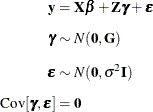The symbols in these expressions denote the following:the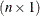vector of responsesthe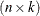design matrix for the fixed effects
the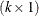vector of fixed-effects parametersthe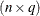design matrix for the random effects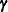the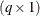vector of random effectsthevector of unobservable residual errors

As is customary for statistical models in the linear mixed model family, the random effects are assumed normally distributed. The same holds for the residual errors and these are furthermore distributed independently of the random effects. As a consequence, these assumptions imply that the response vectorhas a multivariate normal distribution.

Further assumptions, implicit in the preceding expression, are as follows:

• The conditional mean of the data—given the random effects—is linear in the fixed effects and the random effects.

• The marginal mean of the data is linear in the fixed-effects parameters.